MySQL Workbench

## 7.5 Tutorial: Using Explain to Improve Query Performance

This tutorial describes how to use Explain reports to locate and fix problematic (slow) queries. It uses the DBT-3 database and begins with the following simple query example.

```  SELECT * FROM orders
WHERE YEAR(o_orderdate) = 1992 AND MONTH(o_orderdate) = 4
AND o_clerk LIKE '%0223';
```

As shown in the figure that follows, the query example was first executed in the Visual SQL editor. Next, an Explain report was generated by clicking Query and then Explain Current Statement from the menu. The initial report shows a Visual Explain image with information that appears when you move your pointer device over the `orders` table in full table scan.

Figure 7.8 DBT-3 Explain Tutorial: Visual Explain with Full Table Scan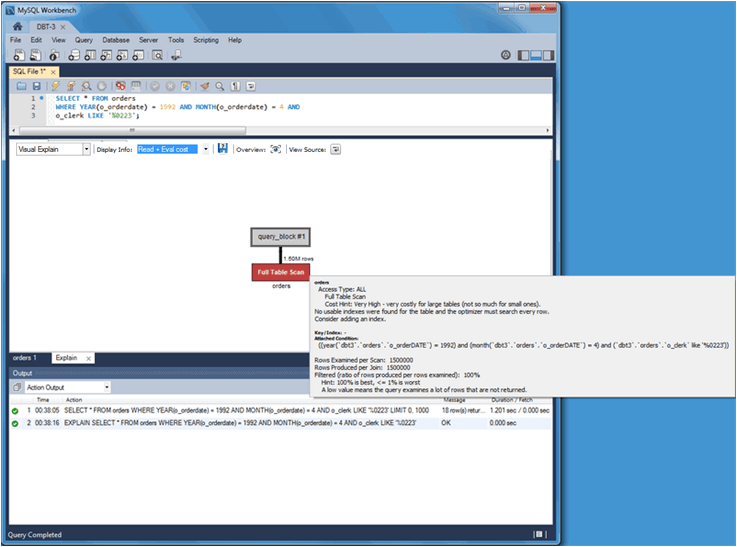Optionally, you can switch to Tabular Explain as the next figure shows. Use the drop-down list to switch between the visual and tabular representations.

Figure 7.9 DBT-3 Explain Tutorial: Tabular Explain with Full Table Scan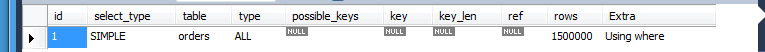• Why did this query generate a full table scan?

• Why is the indexed `o_orderdate` column missing as a possible key?

Looking more closely, also notice that the indexed column is being used in an expression as ```"WHERE YEAR(o_orderdate) = 1992 AND MONTH(o_orderdate) = 4"```, so the index is not used. To use the existing index, you can adjust the query as follows.

```  SELECT * FROM orders
WHERE o_orderdate BETWEEN '1992-04-01' AND '1992-04-30'
AND o_clerk LIKE '%0223';
```

The updated query example results in a Visual Explain image in which `Index Range Scan` replaces the ```Full Table Scan``` generated by the last query example. The next two figures show the visual and tabular representations of the modified query example.

Figure 7.10 DBT-3 Explain Tutorial: Visual Explain with Index Range Scan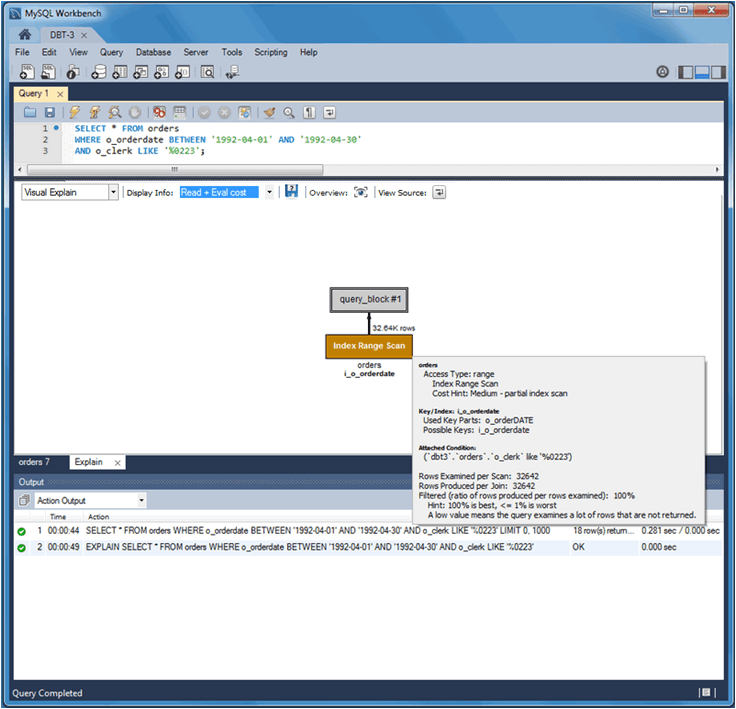Figure 7.11 DBT-3 Explain Tutorial: Tabular Explain with Index Range Scan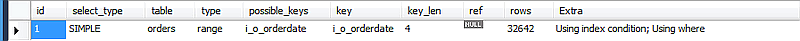Notice the differences. The Type changed from `ALL` to `range`, possible keys (and used key) changed from `NULL` to `i_o_orderdate`, and the number of scanned rows changed from 1.5 million to about 33 thousand. Still, scanning 33 thousand rows while returning just 18 is unnecessary, so the focus can shift to the `o_clerk` column. The next query example (and Tabular Explain figure) adds the following index that should improve performance.

```CREATE INDEX i_o_clerk ON orders(o_clerk);
```

Figure 7.12 DBT-3 Explain Tutorial: Tabular Explain with Index Range Scan and After IndexThe new index is not being considered as a possible key because the query is searching the suffix of the `o_clerk` column and indexes do not work with suffixes (although they do work with prefixes). Instead, this simple example could use the entire clerk ID. Adjusting the query as follows shows better results.

```SELECT * FROM orders
WHERE o_orderdate BETWEEN '1992-04-01' AND '1992-04-30'
AND o_clerk LIKE 'Clerk#000000223';
```

The figures that follow represent the effect of the updated query example in Visual Explain and Tabular Explain respectively.

Figure 7.13 DBT-3 Explain Tutorial: Visual Explain with Index Range Scan and Full ID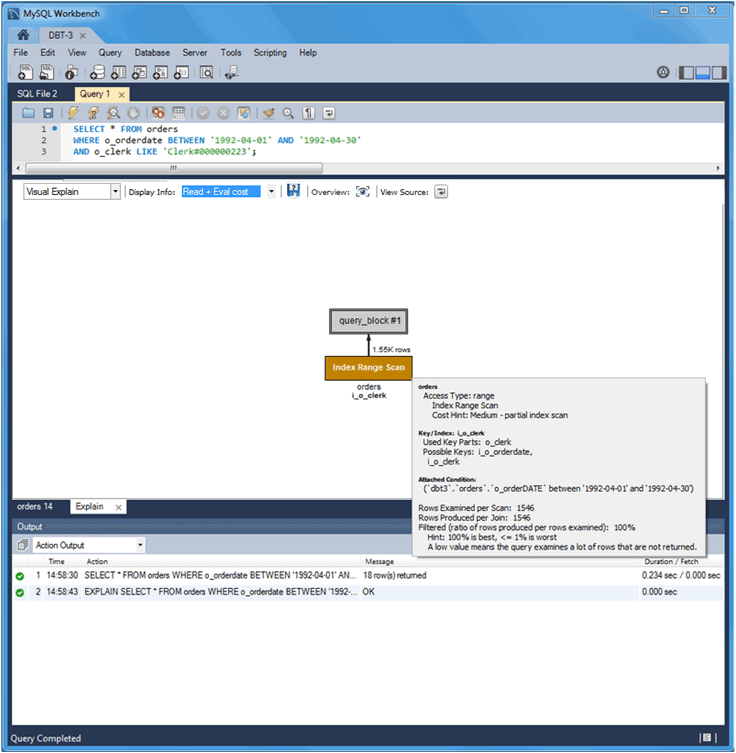Figure 7.14 DBT-3 Explain Tutorial: Tabular Explain with Index Range Scan and Full ID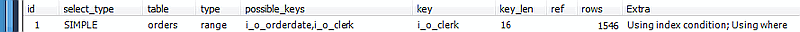The new `o_clerk` index was considered and used, and the query scanned 1546 rows instead of 32642, and the query execution improved from 0.281 to 0.234 seconds. However, `EXPLAIN` estimates that this query scans 1546 rows to return 18. After reviewing the query again, consider that a multiple-column index can meet the conditions of the `WHERE` clause that is based on both the `o_orderdate` and `o_clerk` columns as the next statement shows.

```CREATE INDEX io_clerk_date ON orders(o_clerk, o_orderdate)
```
Note

`o_clerk` appears as the first column in the index because `o_orderdate` uses a range.

Now, executing the adjusted query produces even better results. An estimated 18 rows are both scanned and returned, and the execution time of the query example is 0.234 seconds as the next Visual Explain and Tabular Explain figures show.

Figure 7.15 DBT-3 Explain Tutorial: Visual Explain with Multiple-Column Index Range Scan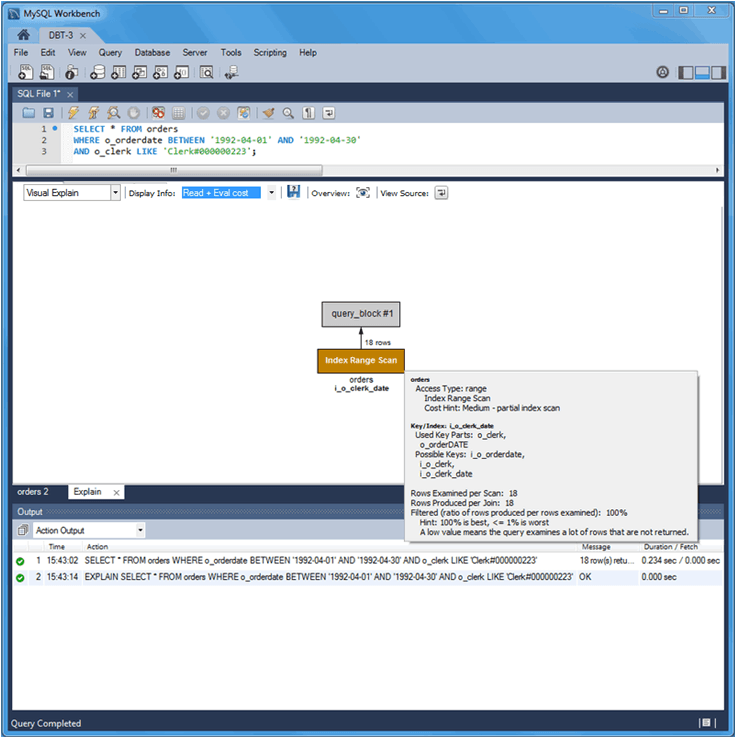Figure 7.16 DBT-3 Explain Tutorial: Tabular Explain with Multiple-Column Index Range Scan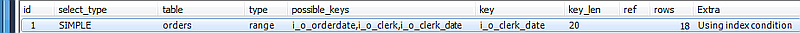The table that follows summarize the results of the modifications made to the query during this tutorial.

Table 7.2 DBT-3 Explain Tutorial Query Comparison

TypePossible keysKeyRows ScannedDuration (seconds)Extra infoRows returned
allNULLNULL1.50M1.201Using where18
rangei_o_orderdatei_o_orderdate326420.281Using index condition; Using where18
rangei_o_orderdate, i_o_clerki_o_clerk15460.234Using index condition; Using where18
rangei_o_orderdate, i_o_clerk, i_o_clerk_datei_o_clerk_date180.234Using index condition18